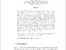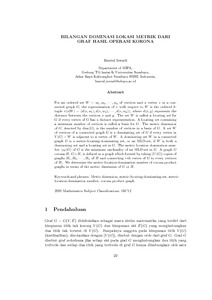# Bilangan Dominasi Lokasi Metrik dari Graf Hasil Operasi Korona

Iswadi, Hazrul (2011) Bilangan Dominasi Lokasi Metrik dari Graf Hasil Operasi Korona. Prosiding Seminar Nasional Matematika Unand 2011. pp. 22-29. ISSN 978-602-19249-0-7Preview PDF hazrul_Bilangan Dominasi Lokasi Metrik_2011.pdf - Published Version Download (190kB) | Preview

## Abstract

For an ordered set $W = {w_1, w_2 , \cdots, w_k}$ of vertices and a vertex $v$ in a connected graph $G$, the representation of $v$ with respect to $W$ is the ordered $k$-tuple $r(v|W) = (d(v,w_1), d(v,w_2 ), \cdots, d(v,w_k))$, where $d(x,y)$ represents the distance between the vertices $x$ and $y$. The set $W$ is called a locating set for $G$ if every vertex of $G$ has a distinct representation. A locating set containing a minimum number of vertices is called a basis for $G$. The metric dimension of $G$, denoted by dim($G$), is the number of vertices in a basis of $G$. A set $W$ of vertices of a connected graph $G$ is a dominating set of $G$ if every vertex in $V(G) - W$ is adjacent to a vertex of $W$. A dominating set $W$ in a connected graph $G$ is a metric-locating-dominating set, or an MLD-set, if $W$ is both a dominating set and a locating set in $G$. The metric location domination number $\gamma_M(G)$ of $G$ is the minimum cardinality of an MLD-set in $G$. A graph $G$ corona $H$, $G \odot H$, is defined as a graph which formed by taking $|V(G)|$ copies of graphs $H_1, H_2, \cdots, H_n$ of $H$ and connecting $i$-th vertex of $G$ to every vertices of $H_i$. We determine the metric-location-domination number of corona product graphs in terms of the metric dimension of $G$ or $H$.

Item Type: Article Metric dimension, metric-locating-dominating set, metric-location-domination number, corona product graph. Q Science > QA Mathematics Academic Department > Department of Mathematics and Natural Science Hazrul Iswadi 6179 29 Mar 2012 05:38 24 Mar 2021 14:17 http://repository.ubaya.ac.id/id/eprint/273View Item